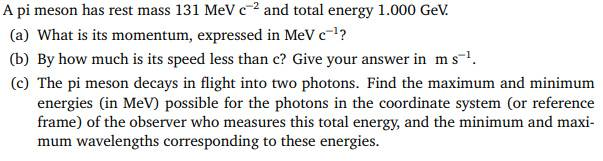# Relativistic momentum of two photons from a decay

## Homework Statement## Homework Equations

(1) E2 = p2c2 + m02c4

(2) E = γm0c2

(3) E = Eγ1 - Eγ2

(4) p = E / c

(5) E = hf

(6) λ = c / f

## The Attempt at a Solution

a) Using eqn (1), rearranged p = (E - m0c2) / c , I obtained 2.9 MeV c-1. Not sure if I have the right answer here as I converted the 1.000 GeV to 1*103 MeV and kept both the rest energy and total energy in mega electron volts.

b) Using eqn (2), rearranged γ = E / m0c2 , γ = 7.63. Then by approximation of the lorentz factor I obtained a difference in velocity to be 2.57*106 ms-1

c) Is where I am stuck. From the observers reference frame or the labs reference frame, the two photons would be in different directions. So by using eqn (3) some how find the two different energies?
And then I guess by using eqns (5) & (6) you can find the wavelength? Bit stuck on the mathematics here.

Thanks for any help and happy holidays :).

#### Attachments

Andrew Mason
Homework Helper
c) Is where I am stuck. From the observers reference frame or the labs reference frame, the two photons would be in different directions. So by using eqn (3) some how find the two different energies?
And then I guess by using eqns (5) & (6) you can find the wavelength? Bit stuck on the mathematics here.
The energies of the photons have to be divided in a way that also conserves momentum. Think of the decay in the pi meson frame. Then in the lab frame.

AM

Hello,

In the pi meson frame then distribution of momentum would be 1/2 for each photon. Does this imply the energy of one of the photons in the lab reference frame would be E' = γE ?

And then with de Broglie's wave-particle duality: λ = h / p , so, λ = hc/E for each wavelegth?

Andrew Mason
Homework Helper
What would the total energy of the photons be in the rest frame of the pi meson? Then assume that the direction of one of the photons is in the direction of motion of the pi meson. Then translate the photon energies to the lab frame.

AM

Andrew Mason